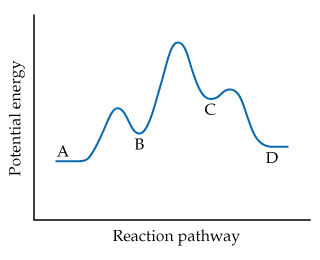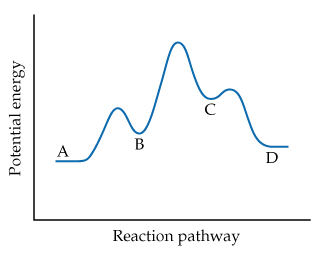Chemistry Practice Problems Energy Diagram Practice Problems Solution: Based on the following reaction profile, how many ...

🤓 Based on our data, we think this question is relevant for Professor Harville's class at UAB.

# Solution: Based on the following reaction profile, how many intermediates are formed in the reaction AD?

###### Problem

Based on the following reaction profile, how many intermediates are formed in the reaction AD?###### Solution

We are asked based on the following reaction profile, how many intermediates are formed in the reaction AD.A reaction profile/diagram is a diagram showing the change in chemical potential energy as the chemical reaction proceeds from reactants to the product.View Complete Written Solution

Energy Diagram

Energy Diagram

#### Q. For the reaction A → D, is ΔE positive, negative, or zero?

Solved • Thu Dec 06 2018 09:35:46 GMT-0500 (EST)

Energy Diagram

#### Q. The gas-phase reaction Cl(g) + HBr(g)HCl(g) + Br(g) has an overall energy change of -66 kJ. The activation energy for the reaction is 7 kJ.What is the...

Solved • Thu Dec 06 2018 09:35:45 GMT-0500 (EST)

Energy Diagram

#### Q. The gas-phase reaction Cl(g) + HBr(g) → HCl(g) + Br(g) has an overall energy change of –66 kJ. The activation energy for the reaction is 7 kJ. Sketch ...

Solved • Thu Dec 06 2018 09:35:45 GMT-0500 (EST)

Energy Diagram

#### Q. Based on their activation energies and energy changes and assuming that all collision factors are the same, which of the following reactions would be ...

Solved • Thu Dec 06 2018 09:35:45 GMT-0500 (EST)